# [PYTHON] What is xg boost (1) (for beginners)

As a data scientist, I would like to look back and organize what I have come to Gamshala. I will not deal with actual actual data, but I will write down what I have learned to produce output. First of all, I want to handle xgboost, but from ensemble learning in the first place. (xgboost is a kind of ensemble learning) Xgboost is a versatile method for predictive tasks.

# What is ensemble learning?

When you want to solve a prediction problem with data, you can combine multiple learning devices to improve accuracy and create one learning device.

The accuracy will increase because the learning device is created based on the result, and several learning devices are created comprehensively, instead of creating only one learning device. The idea. It can be roughly classified into three types. Basic knowledge before that.

### Bias and variance

Bias: Average of actual and predicted values Variance: The degree to which the predicted values are scattered

④ is a state with good accuracy because it has low bias and low variance. ③ is a state of high variance. The model is likely to be overfitting. The accuracy tends to be poor when forecasting using new data. ② is a high bias state. There is a high possibility that the data has not been learned in the first place.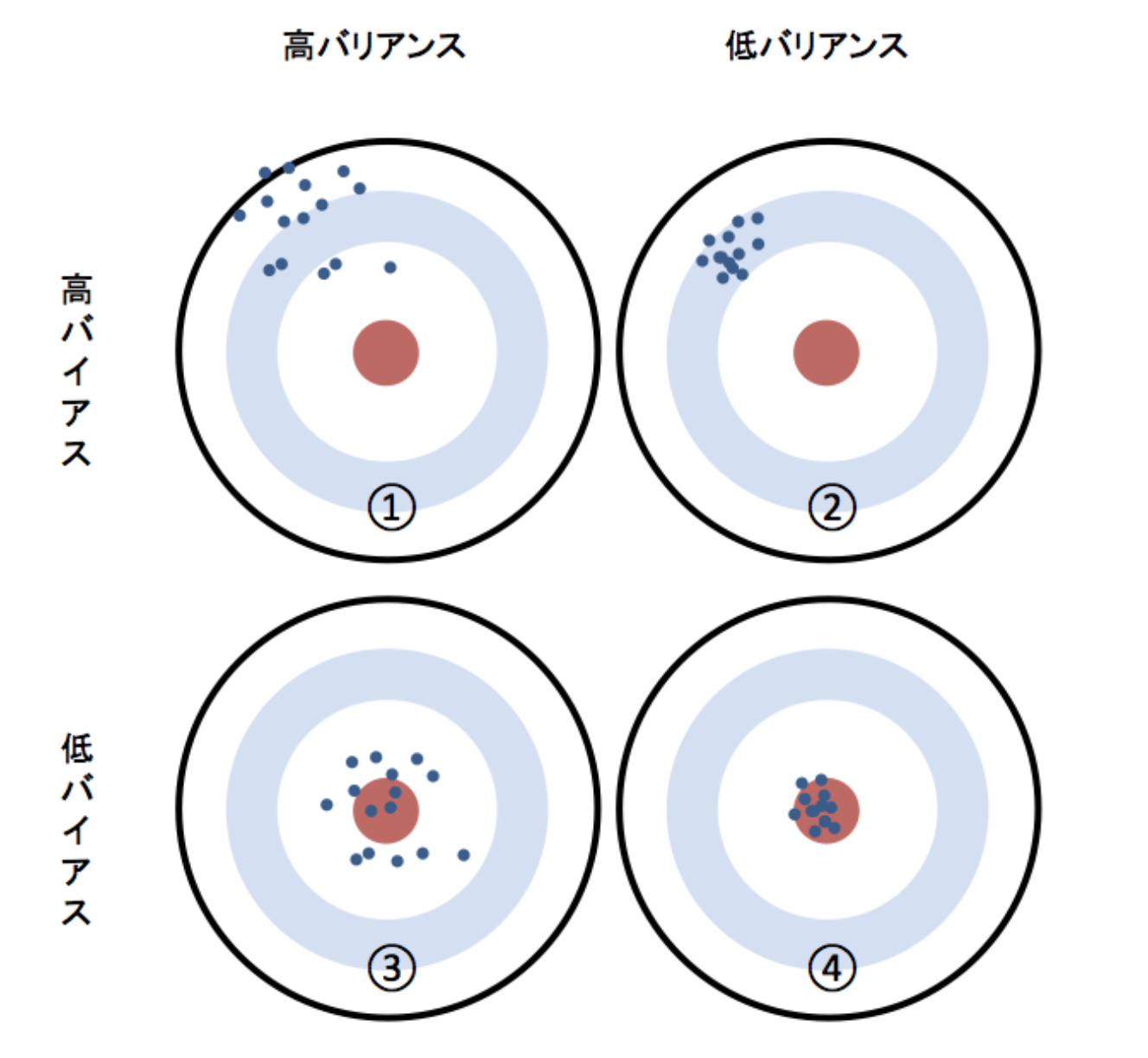The figure is very easy to understand, so I will borrow the figure on the reference page. [Are all advanced machine learning users using it? !! I will explain the mechanism of ensemble learning and the three types] (https://www.codexa.net/what-is-ensemble-learning/)

## Three methods

### Bagging

Generally, it has the characteristic of lowering the variance of the prediction result of the model. In bagging, the data set is made diverse by restoring and extracting the training data using the boost trap method. Restoration extraction is an extraction method in which a sample once extracted is subject to extraction again.

Aggregate each result to create one learner. If it is regression, it is decided by the average value, and if it is classification, it is decided by majority vote.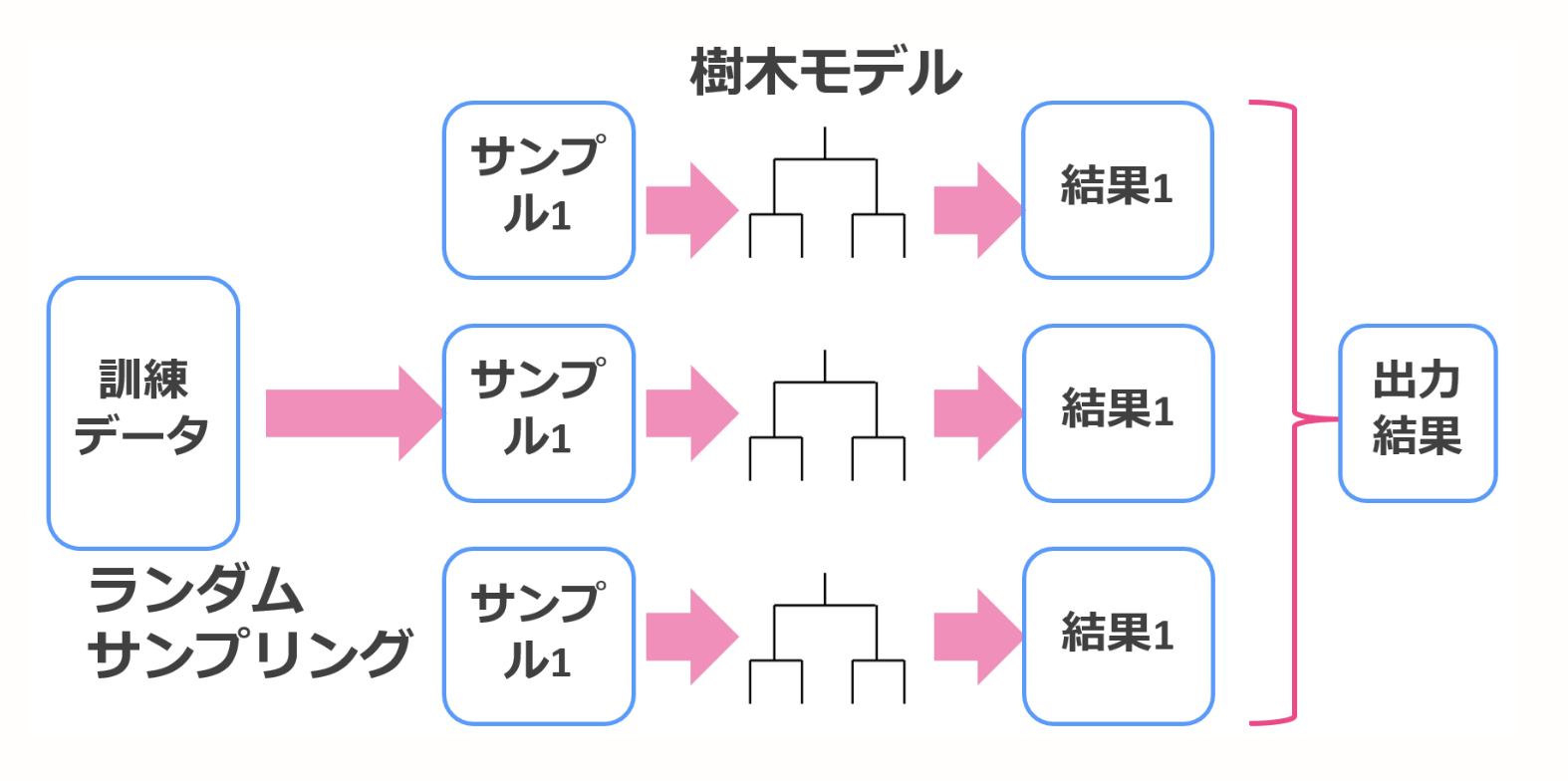The figure is very easy to understand, so I will borrow the figure on the reference page. [What is ensemble learning? Difference between bagging and boosting](https://toukei-lab.com/ensemble)

### Boosting

Generally, it has the characteristic of lowering the bias with respect to the prediction accuracy of the model. Boosting first trains the underlying model to establish a baseline. The basic model used as the baseline is iteratively processed many times to improve the accuracy. Focusing on the wrong prediction of the basic model and adding "weight" to improve the next model. Make a model and make a new model with mistakes. Finally put everything together. xgboost is an implementation that uses this boosting.

It takes time to learn, probably because the first model is taken into consideration.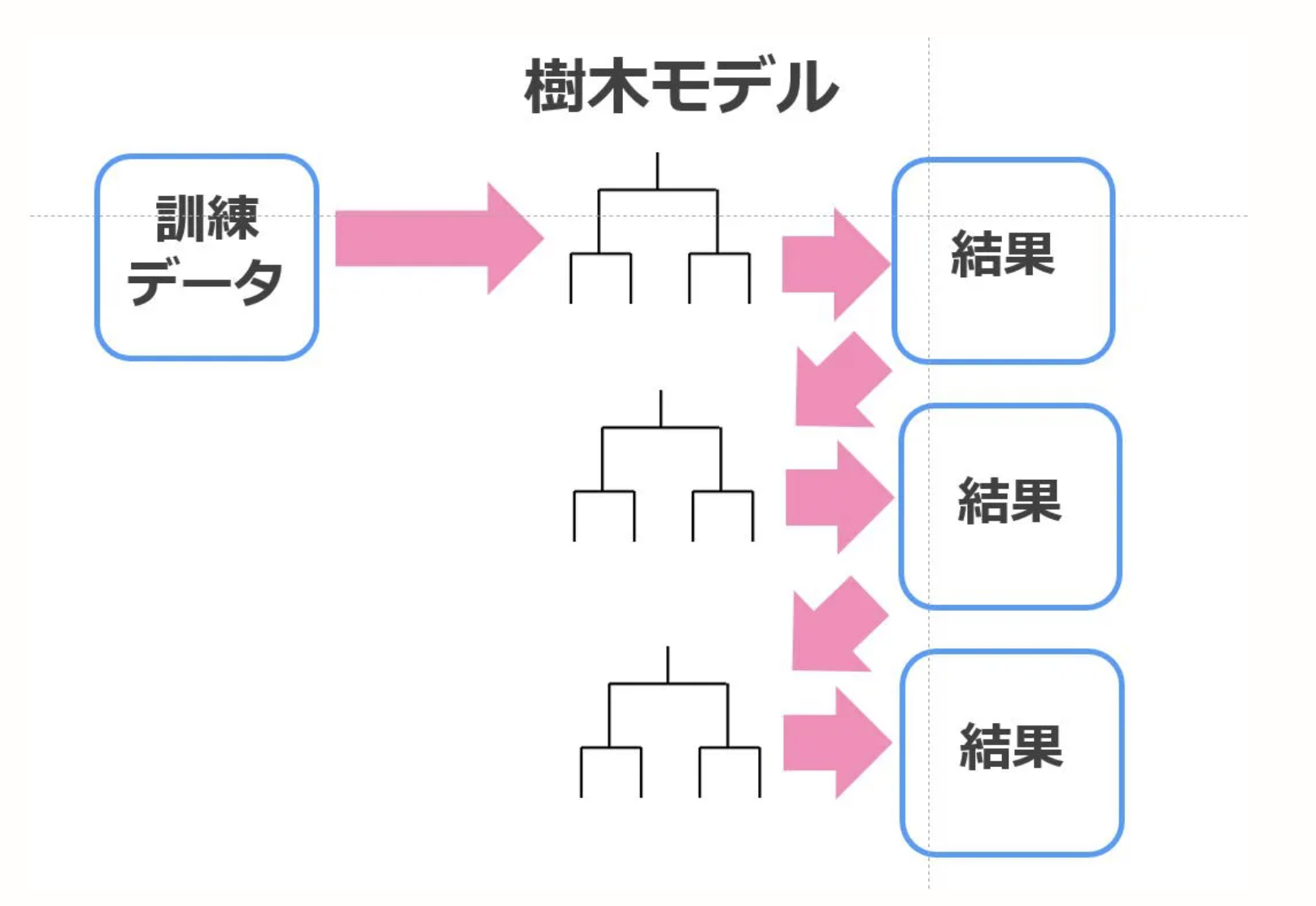The figure is very easy to understand, so I will borrow the figure on the reference page. [What is ensemble learning? Difference between bagging and boosting](https://toukei-lab.com/ensemble)

### Stacking

How to stack models. It seems that it is possible to adjust the bias and variance to incorporate it ... It was complicated, so I will omit it.

A model that learns by looking for a direction that minimizes loss when a function or loss function is defined.

Have an image with a mathematical formula.

## Loss function

A certain index (= function) is used to judge how much the predicted value and the actual value are, and whether there is a difference. Move the model parameters so that the index is minimized.

The loss function of XGBoost is as follows.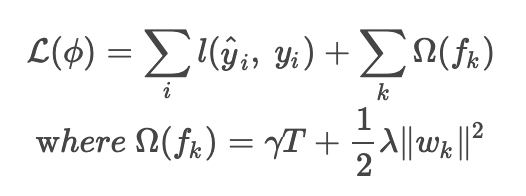The sum of the errors between the predicted value and the target value plus the regularization term.

The second equation represents the regularization term. The presence of the regression tree weight w is taken into account by w when minimizing the loss function and prevents overfitting.

The explanation of each variable is as follows.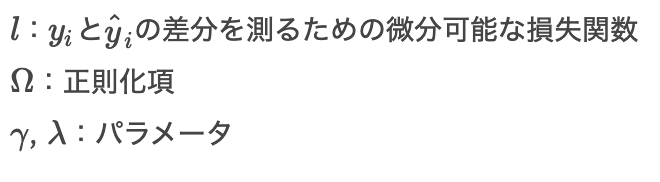## Function optimization

Now that we have a loss function, all we have to do is optimize it.

When the above loss function is transformed and differentiated by w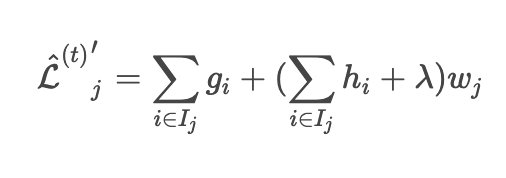Will be.

When this equation is 0, w minimizes the loss function. (High school math level)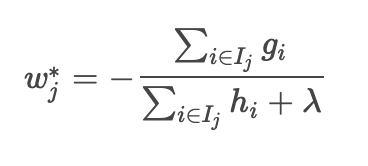Will be.

Substituting this into an approximate loss function gives the minimum loss value. Here, q the structure of XGBoost.This is the formula that evaluates xgboost.

## Move the sample

Use a breast cancer dataset.

``````import xgboost as xgb
import numpy as np
from sklearn import datasets
from sklearn.model_selection import train_test_split
from sklearn.metrics import accuracy_score

def main():
X, y = dataset.data, dataset.target
#Split for learning and verification
X_train, X_test, y_train, y_test = train_test_split(X, y,
test_size=0.3,
shuffle=True,
random_state=42,
stratify=y)
#Change the format of the dataset
dtrain = xgb.DMatrix(X_train, label=y_train)
dtest = xgb.DMatrix(X_test, label=y_test)
#Parameters for learning
xgb_params = {
#Binary classification
'objective': 'binary:logistic',
#Evaluation index
'eval_metric': 'logloss',
}
#Learn with xgboost
bst = xgb.train(xgb_params,
dtrain,
num_boost_round=100,
)
#Calculated with verification data
y_pred_proba = bst.predict(dtest)
#Threshold 0.5 to 0,Convert to 1
y_pred = np.where(y_pred_proba > 0.5, 1, 0)
#See accuracy
acc = accuracy_score(y_test, y_pred)
print('Accuracy:', acc)

if __name__ == '__main__':
main()
``````

result

``````acc: 0.96
``````

## Summary

xgboost is a model that creates and learns a model by boosting, and determines and updates parameters with gradient information during learning.